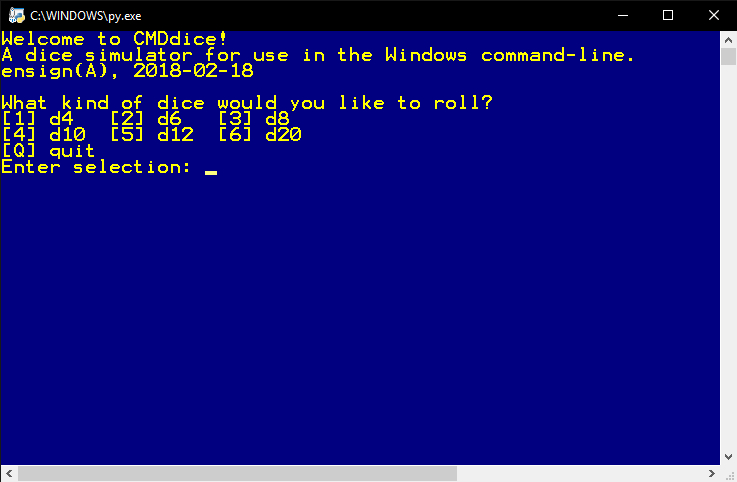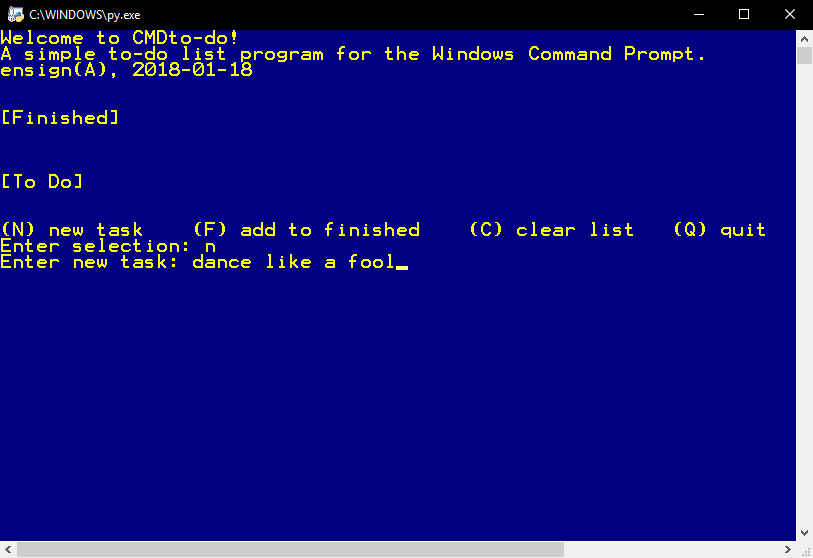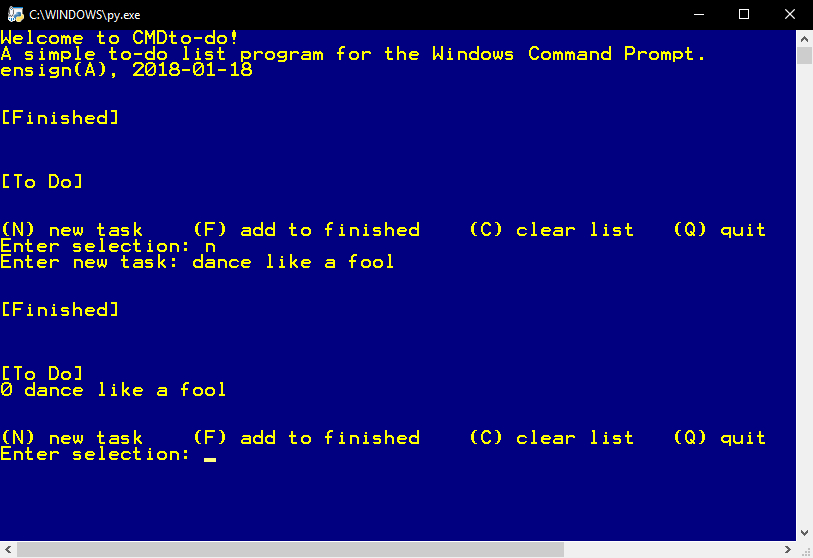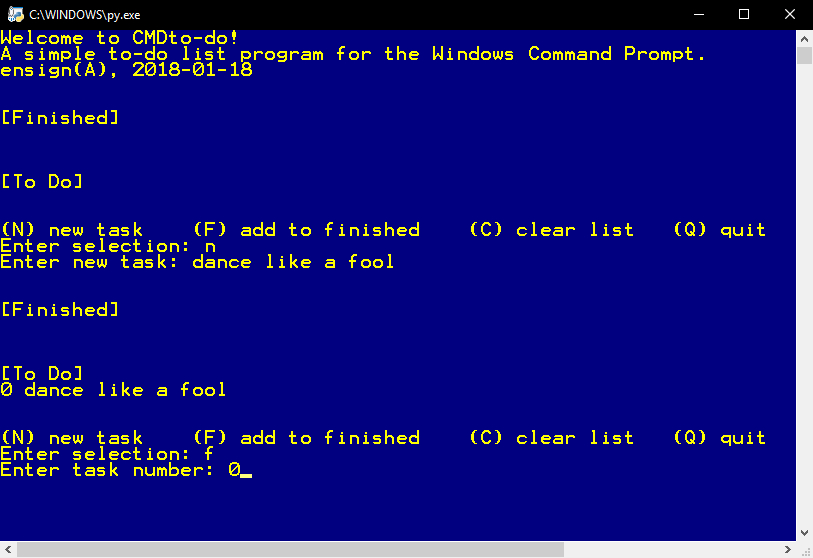Main Page

# CMDdice():

A dice rolling simulator for the Windows command-line.

This is an app I made to use on my laptop during Dungeons and Dragons sessions. I normally use real dice but it's nice to have a backup plan and the real point of making this was to practice coding in Python.^ This is the main screen. Like many terminal based apps selections are made by hitting an indicated key and then enter, or entering text for the list content.

|^ We will select the number of sides we want our dice to have and how many dice we want to roll.

|^ The elves in the computer will shake the dice, and...

|^ ...the results are in! The program prints what each die rolled and the total sum. One shortcoming of this program is that you cant mix dice types in one roll- perhaps that will come in another version.

|

## Sourcecode()

``````
# name: ensign(A)
# date: 2018-02-18
# description: A dice simulator for use in the Windows command-line.
# version 1.0

import random
import time
import sys

def error():
print('')
print('Invalid selection.')
print('')

def goodbye():
print('Goodbye!')
time.sleep(2)
sys.exit()
#program ends

def selectdice():
#type of dice
print('What kind of dice would you like to roll?')
print(' d4    d6    d8')
print(' d10   d12   d20')
print('[Q] quit')
choice = input('Enter selection: ')
dice = 0
if choice == '1':
dice = 4
elif choice == '2':
dice = 6
elif choice == '3':
dice = 8
elif choice == '4':
dice = 10
elif choice == '5':
dice = 12
elif choice == '6':
dice = 20
elif choice == 'q' or choice == 'Q':
goodbye()
else:
error()
main()

return dice

def dicenum():
#number of dice
print('How many dice would you like to roll?')
print('Limit = 10')
n = (input("# of dice: "))
try:
num = int(n)
if (num > 10) or (num < 1):
print('')
print('Enter a number between 1 and 10.')
print('')
dicenum()
else:
return num
except:
print('')
print('That is not a number.')
print('')
dicenum()

def roll_loop(dice, num):
rloop = 0
total = 0
time.sleep(1)
print('shake shake')
time.sleep(1)
print('shake shake')
time.sleep(1)
print('roll!')
time.sleep(2)
#rolls dice the number of times specified
while(rloop < num):
rloop = (rloop + 1)
n = random.randint(1, dice)
total = (total + n)
print('rolled ', n)
print('')
print("The result is:", total)
print('')
time.sleep(1)
rollagain()

def result(total):
#result message
time.sleep(1)
print('shake shake')
time.sleep(1)
print('shake shake')
time.sleep(1)
print('roll!')
time.sleep(2)
print("The result is:", total)
print('')
time.sleep(2)

def rollagain():
#restart/quit
print('')
print('Would you like to roll again?')
print('[Y] yes  [N] no')
choice = input('Enter selection: ')

if choice == 'y' or choice == 'Y':
main()

elif choice ==  'n' or choice == 'N':
goodbye()

else:
error()
rollagain()

def main():
#main program
roll_loop(selectdice(), dicenum())
rollagain()

#welcome message outside loop so it only shows once
print('Welcome to CMDdice!')
print('A dice simulator for use in the Windows command-line.')
print('ensign(A), 2018-02-18')
print('')

main()
``````